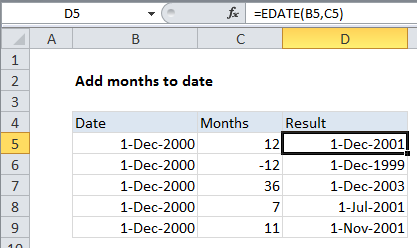## Excel Office

Excel How Tos, Tutorials, Tips & Tricks, Shortcuts

# Add months to date in Excel

It you need to add months to date in Excel then this tutorials is for you. See example below:

To add a given number of years to a date, you can use the EDATE function.

## Formula

`=EDATE(date,months)`## Explanation

In the example shown, the formula in D5 is:

`=EDATE(B5,C5)`

### How this formula works

The EDATE function is fully automatic. Simply supply a valid date and a number of months and EDATE will return a new date. To subtract months from a date, supply a negative value.

Also See:   How to get same date next year or previous year in Excel

```=EDATE(A1,12*10) // 10 years